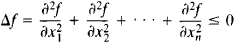# Superharmonic Function

## superharmonic function

[¦sü·pər·här′män·ik ′fəŋk·shən]
(mathematics)
A continuous complex function ƒ whose value at a point z0 exceeds its average values computed by the integral of ƒ around a circle centered at z0.

## Superharmonic Function

a function f(x1, x2,...,xn) that satisfies in some domain the inequality(see).

Mentioned in ?
References in periodicals archive ?
(ii) Let h be a positive superharmonic function in D.
Let [Mathematical Expression Omitted] denote the regularized reduced function (or balayage) of a positive superharmonic function s on [R.sup.n] relative to a set S.

Site: Follow: Share:
Open / Close